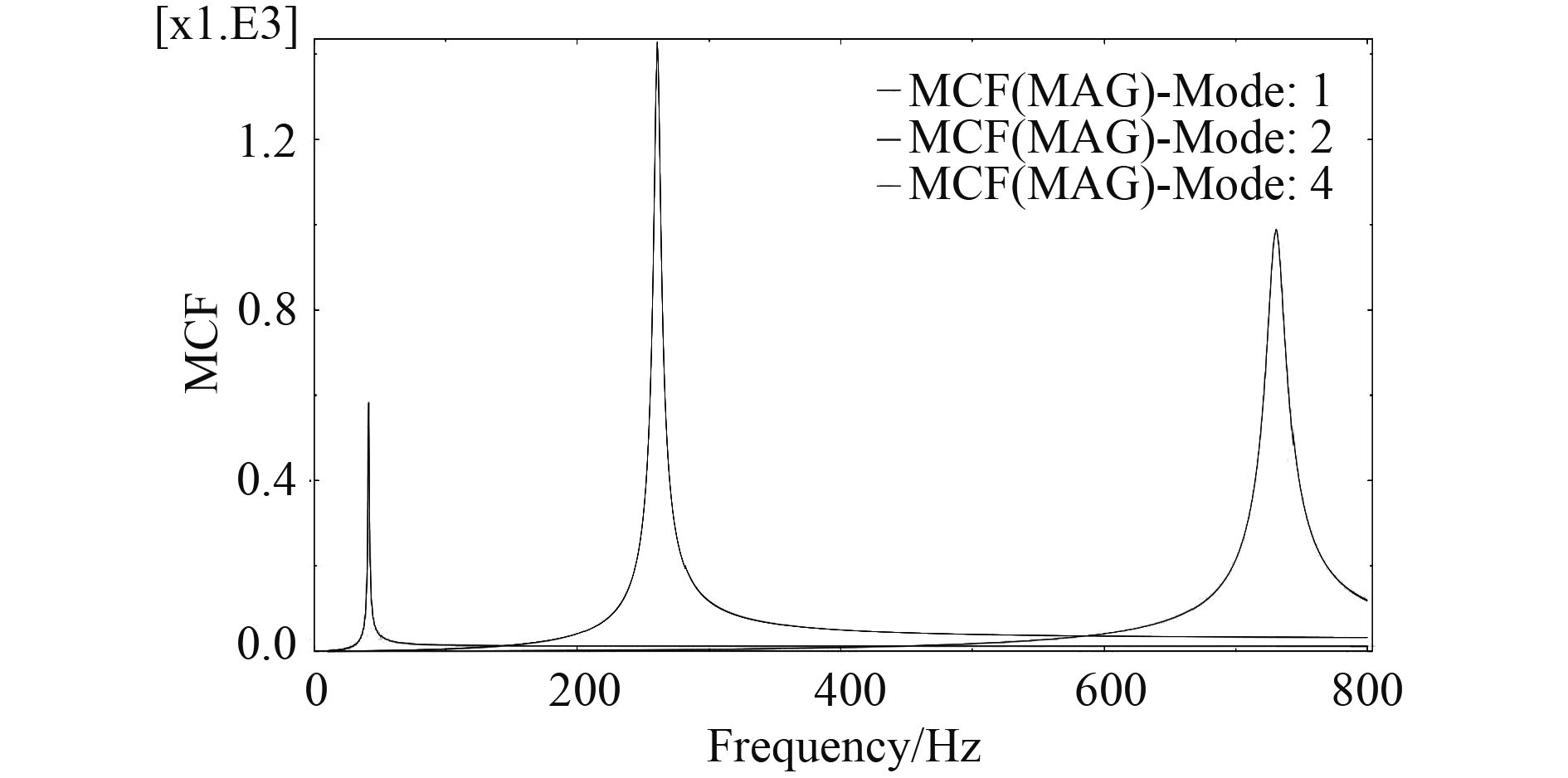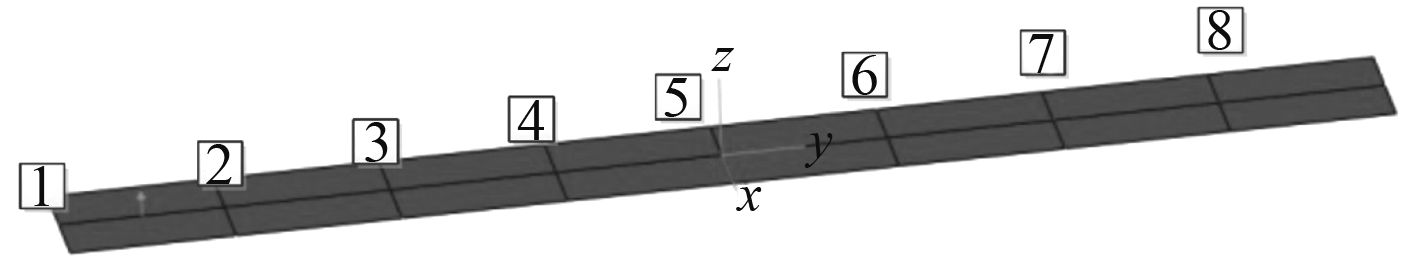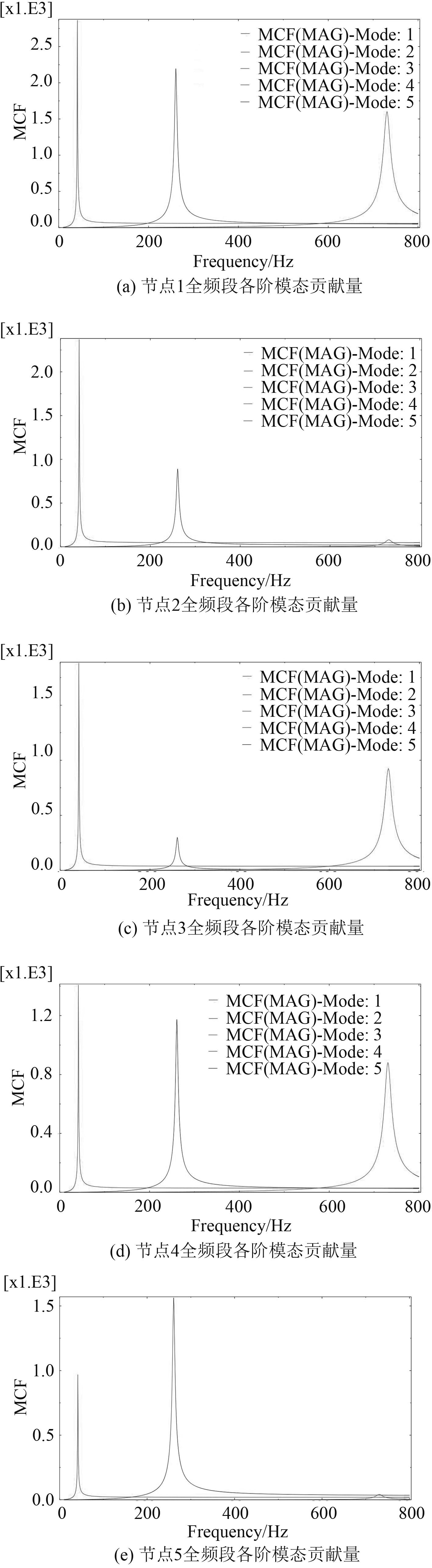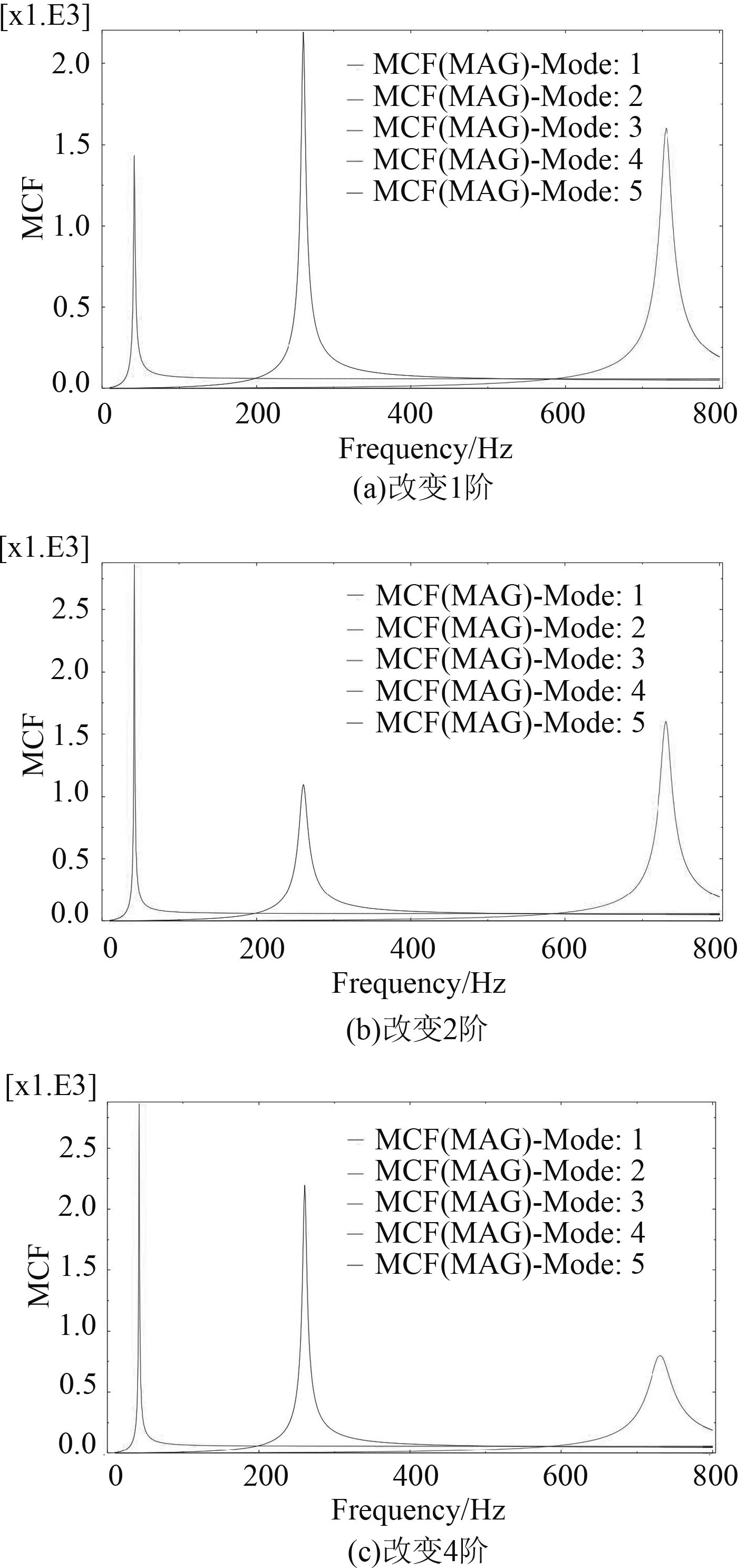﻿ 结构振动响应优势模态选取方法研究
 舰船科学技术2018, Vol. 40Issue (4): 43-47PDF

Research on selection method of dominant modes in structure vibration response
LI Hua-dong, WANG Yong-li, MEI Zhi-yuan
Department of Naval Architecture Engineering, Naval University of Engineering, Wuhan 430033, China
Abstract: Based on modal contribution factors plug in Abaqus/ Standard, the method of choosing the dominant mode of structural vibration response is studied. First, through the characteristic analysis of frequency domain curve of modal contribution factors, two methods of frequency domain peak method and full band method are proposed. In addition, by analyzing the vibration performance and modal contribution of the cantilever beam, according to the above two methods to select the dominant mode respectively, and the effect of the optimized dominant mode damping ratio on the structural vibration suppression is compared, and it is found that the optimal modal damping ratio obtained by full-band method is more effective in reducing the overall vibration response. Finally, the influence of modal displacement and other factors on modal contribution is discussed.
Key words: modal contribution factors     dominant mode     vibration response     optimization analysis
0 引　言

1 基于Abaqus/Standard的优势模态选取方法研究 1.1 模态贡献量在Abaqus/Standard中的应用

1）场输出的每一个固有频率分析步里必须包括节点位移。此外，如果进行的是声固耦合分析，场输出的每个固有频率分析步必须包括节点孔隙或声压。

2）每个固有频率分析步后，必须至少有一个模态叠加的稳态动力学分析步（不可以使用直接法或子空间法）。

3）在每个模态叠加稳态动力学分析步里，历史输出里必须包括广义位移和广义位移的相位角。

1.2 优势模态的选取方法研究

 $P(\bar x;\varOmega ) = \sum\limits_{\alpha = 1}^N {{p_\alpha }(\bar x;\varOmega )} = \sum\limits_{\alpha = 1}^N {{\phi _\alpha }(\bar x){q_\alpha }(\varOmega )} \text{，}$ (1)图 1 模态贡献量频域曲线 Fig. 1 Frequency of domain curves of MCF

1）峰值法

 \begin{aligned}{p_\alpha }({x_A};\varOmega ) =& \sum\limits_{m = 1}^M {{p_{\alpha m}}({x_A};\varOmega )} = \sum\limits_{m = 1}^M {{\phi _{\alpha m}}({x_A}){q_\alpha }(\varOmega )} \text{，}\\& MC{F_\alpha }_{({x_A})} = \frac{{{p_\alpha }({x_A};\varOmega )}}{{\sum\limits_{\alpha = 1}^N {{p_\alpha }({x_A};\varOmega )} }}\text{。}\end{aligned} (2)

2）全频段法

 \begin{aligned}{p_\alpha }(\bar x;\varOmega ) =& \sum\limits_{m = 1}^M {{p_{\alpha m}}(\bar x;\varOmega )} = \sum\limits_{m = 1}^M {{\phi _{\alpha m}}(\bar x){q_\alpha }(\varOmega )} \text{，}\\&MC{F_\alpha }_{(\bar x)} = \frac{{{p_\alpha }(\bar x;\varOmega )}}{{\sum\limits_{\alpha = 1}^N {{p_\alpha }(\bar x;\varOmega )} }}\text{。}\end{aligned} (3)
2 两种优势模态对整体动态响应的影响分析

2.1 算例模型图 2 算例模型 Fig. 2 Model of case

2.2 基于模态贡献量的优化设计及结果分析 2.2.1 模型的前800 Hz频段内固有频率及振型表 1 仿真模型1至5阶振型 Tab.1 1st to 5th vibration mode of simulation
2.2.2 模型模态贡献量的计算1）峰值法表 2 各模态所有节点的模态贡献量峰值 Tab.2 Peak value of MCF of output node

①由于第3阶是X-Y水平面的弯曲振型，第5振型是沿模型Y轴中线的扭转振型，模型Y轴中线的模态幅值为0，全部为此模态振动的驻点，而激励点也在模型的Y轴中线，根据式（2），激励在这2阶的模态力为0，无法激起此振型，因此，第3阶、第5阶的模态贡献量为0；

②在1阶模态中，各个节点的振型位移随着节点编号的增加而减小，而其模态贡献量的峰值同样随之减小。同样，2阶、4阶的各个节点模态贡献量峰值的变化趋势与节点所在的模态振幅变化趋势相同。

2）全频段法表 3 全频段内各模态所有节点的模态贡献量 Tab.3 MCF of output node in full-band

2.2.3 两种优势模态对整体振动响应影响分析表 4 改变模态阻尼比后的总加速度级 Tab.4 Acceleration level after changing modal damping ratio图 4 改变各阶模态阻尼比后节点1各阶模态贡献量 Fig. 4 First 5 vibration modes’ MCF of 1st output node after changing modal damping ratio

4阶模态为Z向2个周期的弯曲振动，因此可以考虑在结构合理的位置增加抑制Z向弯曲振动的阻尼材料，同时1阶和2阶分别是Z向1个周期的弯曲振动和Z向1.5个周期的弯曲振动，Z向弯曲振动的阻尼材料同样可以抑制1阶模态和2阶模态。

3 结　语

1）增加由 $MC{F_\alpha }_{(\bar x)}$ 所得出优势模态的模态阻尼比，其结构整体振动响应降低最多，优化效率更高，振动响应变化量的相对大小与 $MC{F_\alpha }_{(\bar x)}$ 相同；

2）增加由 $MC{F_\alpha }_{({x_A})}$ 所得出优势模态的模态阻尼比，可以有效地降低结构振动的响应峰；

3）如果某一阶的模态贡献量所占比重为0，则改变其模态阻尼比不会对振动响应有影响；

4）不管是峰值法或是全频段法，各个节点模态贡献量的变化趋势与节点所在的模态振幅变化趋势相同。

  陈志坚. 舰艇振动学[M]. 北京: 国防工业出版社, 2010: 1–5.  宋文, 梁跃, 高爱军, 等. 基于模态贡献量法支撑结构振动控制方法[J]. 鱼雷技术, 2015(6): 439–443. SONG Wen, LIANG Yue, GAO Ai-jun, et al. Vibration control of engine support structure based on modal contribution method[J]. Torpedo Technology, 2015(6): 439–443.  DU Hua-jun, YU Bai-sheng. Vibration suppression of a conical honeycomb satellite adapter subjected to constrained layer damping[J]. Chinese Journal of Applied Mechanics, 2003, 20(3): 5–9.  DU Hua-jun, HUANG Wen-hu, ZOU Zhen-zhu. Passive vibration control of aerospace supporter [J]. Chinese Journal of Applied Mechanics, 2002, 19(3): 10–13. https://www.researchgate.net/publication/296400684_Passive_vibration_control_of_aerospace_supporter  梁静. 车体模态贡献分析及其对振动影响研究[D]. 成都: 西南交通大学, 2011.  傅志方, 华宏星. 模态分析理论与应用[M]. 上海: 上海交通大学出版社, 2000: 73–107.  沃德·海伦, 等. 模态分析理论与实验[M]. 北京: 北京理工大学出版社, 2001: 2–25.  曹树谦, 张文德, 萧龙翔. 振动结构模态分析理论、实验与应用[M]. 天津: 天津大学出版社, 2002: 181–236.  AUWERAER V H, LEURIDAN J. Identification of structural parameters from dynamic response data[M], 1978: 165–174.